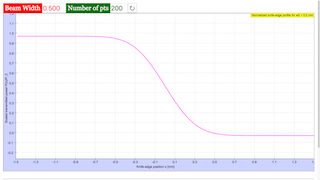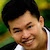Intro Page

Laser Beam Profile

Developed by E. Behringer

This set of exercises guides the student to model the results of an experiment to determine the profile of a laser beam using a knife-edge technique. It requires the development of the model of the knife-edge profile, and fitting of the model profile to experimental data. Here, the computational tasks are handled by built-in functions of the computational tool being used to complete these exercises.

 Subject Area Waves & Optics Beyond the First Year Python Students who complete this set of exercises will be able to express an equation predicting the profile of a laser oscillating in the TEM00 mode in terms of dimensionless (“scaled”) variables suitable for coding (Exercise 1); produce both line plots and contour plots of the (scaled) irradiance of the beam versus (scaled) position(s) (Exercises 1 and 2); develop a model of, and plot, the knife-edge profile of the laser beam (Exercise 3); and fit the model, i.e., of the irradiance versus knife-edge position, to experimental data (Exercise 4) 120 min
Exercise 3

### EXERCISE 3: THE KNIFE-EDGE PROFILE

You can experimentally determine the value of the parameter  that describes the width of the beam by measuring the profile of the laser beam using a knife-edge mounted on a linear translation stage together with an optical detector connected to a power meter. The idea is that you allow the laser beam to enter the detector while you translate the knife-edge across the beam and monitor the power meter reading. Initially, when the beam is not blocked, the reading gives the total power of the beam; finally, the beam is completely blocked from entering the detector by the knife-edge and the reading would be zero (in the absence of background light). A schematic is shown below; we assume the beam is centered on the origin.

Imagine that the knife-edge travels in the -direction, and that the position of the knife-edge is . Then the power received by the detector is

where  is the total power of the laser beam. Show that this expression is equivalent to

where  is the error function. Calculate  and plot this quantity versus , the position of the knife edge, when  mm and for .

Beam_Profile_Exercise_3.py

#

# Beam_Profile_Exercise_3.py

#

# This file will generate a plot of

# the predicted knife-edge beam profile

# for the TEM00 laser mode

#

# Written by:

#

# Ernest R. Behringer

# Department of Physics and Astronomy

# Eastern Michigan University

# Ypsilanti, MI 48197

# (734) 487-8799

# This email address is being protected from spambots. You need JavaScript enabled to view it.

#

# 20160614 by ERB

#

# import the commands needed to make the plot and fit the data

from pylab import xlim,xlabel,ylim,ylabel,grid,show,plot,title

from numpy import linspace,sqrt

from scipy.special import erf

# inputs

npts = 200 # number of intervals in x

w0 = 0.5 # beam width [mm]

# calculated quantity

x_max = 3.0*w0

# set up the array of knife-edge positions

x = linspace(-x_max,x_max,npts+1)

# calculate the scaled power

scaled_P = 0.5*(1.0 - erf(sqrt(2.0)*x/w0))

# Define the limits of the horizontal axis

xlim(-x_max,x_max)

# Label the horizontal axis, with units

xlabel("Knife-edge position $$x$$ [mm]", size = 16)

# Define the limits of the vertical axis

ylim(-0.1,1.1)

# Label the vertical axis, with units

ylabel("Scaled transmitted power $$P(x)/P_0$$", size = 16)

# Make a grid on the plot

grid(True)

# Make the title

title('Normalized knife-edge profile for $$w_0 =$$%s mm'%w0)

# Generate the plot. The line color is magenta (m).

plot(x,scaled_P,"m")

show()

### Translations

Code Language Translator Run### Software Requirements

SoftwareRequirements

 Android iOS Windows MacOS with best with Chrome Chrome Chrome Chrome support full-screen? Yes. Chrome/Opera No. Firefox/ Samsung Internet Not yet Yes Yes cannot work on some mobile browser that don't understand JavaScript such as..... cannot work on Internet Explorer 9 and below

### CreditsFremont Teng; Loo Kang Wee; based on codes by E. Behringer

[text]

[text]

Research

[text]

[text]

[text]

### end faq